# 蒙特卡洛方法在交易策略优化中的应用

13 八月 2018, 09:30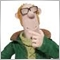0
3 937

### 概率性 EA 模型

1. 经验分布函数用作近似值，

2. 我们使用一个简单的离散分布，其中以交易利润作为两个值中的一个。这是一个亏损概率的平均亏损，或者是一个获利概率的平均利润。

3. 我们构造一个不是离散的，而是连续的（具有密度）近似的分布函数。为此，我们使用例如核密度估计这样的方法。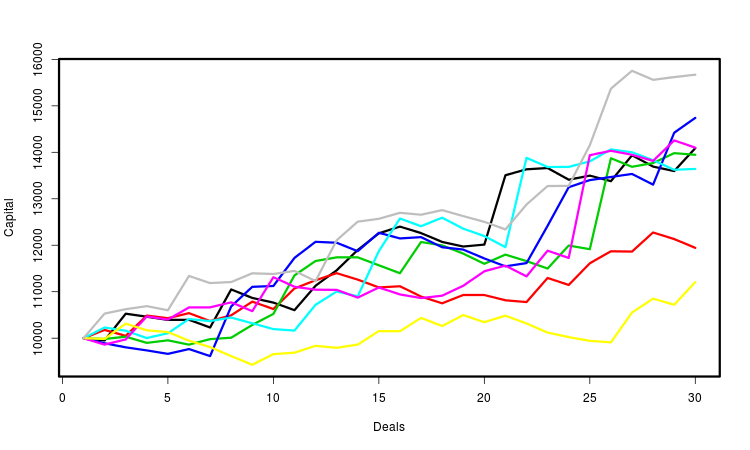### 应用理论

1. 通过蒙特卡洛方法获得了一个可能的利润值的大样本，我们可以研究它的分布和与之相关的数量。这个利润的平均值非常重要，方差也很重要——它越小，EA的工作就越稳定，其未来结果的不确定性也就越小。我们的标准将等于平均利润与平均分散的比率，这与夏普比率类似。
2. 另一个重要的特点是一系列交易中利润的回撤，回撤太大可能会导致存款的亏损，哪怕 EA 是获利的。为此，回撤通常会被约束。了解它会怎样影响到可能的利润很有用，该标准简单地定义为平均利润，但是条件是当超过允许的回撤水平时，交易终止。
3. 我们将建立一个衡量利润的稳定性的持久性的贸易利润分配。从概率论的角度，这意味着交易利润在原则上，价格变化不稳定的时候的 稳定性。为此，我们将会使用类似于前瞻测试的想法。让我们把最初的交易样本分为最初和最终子样本，为了验证它们的同质性，我们可以应用统计学检验。基于这个测试，我们将创建一个优化准则。

`#include <mcarlo.mqh>`

```double OnTester()
{
return optpr();         // 优化参数
}
```

```double optpr()
{
if(noptpr<1||noptpr>NOPTPRMAX) return 0.0;
double k[];
if(!setks(k)) return 0.0;
if(ArraySize(k)<NDEALSMIN) return 0.0;
MathSrand(GetTickCount());
switch(noptpr)
{
case 1: return mean_sd(k);
case 2: return med_intq(k);
case 3: return rmnd_abs(k);
case 4: return rmnd_rel(k);
case 5: return frw_wmw(k);
case 6: return frw_wmw_prf(k);
}
return 0.0;
}
```

setks() 函数根据交易历史计算出交易利润的数组。

```bool setks(double &k[])
{
if(!HistorySelect(0,TimeCurrent())) return false;
uint nhd=HistoryDealsTotal();
int nk=0;
ulong hdticket;
double capital=TesterStatistics(STAT_INITIAL_DEPOSIT);
long hdtype;
double hdcommission,hdswap,hdprofit,hdprofit_full;
for(uint n=0;n<nhd;++n)
{
hdticket=HistoryDealGetTicket(n);
if(hdticket==0) continue;

if(!HistoryDealGetInteger(hdticket,DEAL_TYPE,hdtype)) return false;

hdcommission=HistoryDealGetDouble(hdticket,DEAL_COMMISSION);
hdswap=HistoryDealGetDouble(hdticket,DEAL_SWAP);
hdprofit=HistoryDealGetDouble(hdticket,DEAL_PROFIT);
if(hdcommission==0.0 && hdswap==0.0 && hdprofit==0.0) continue;

++nk;
hdprofit_full=hdcommission+hdswap+hdprofit;
k[nk-1]=1.0+hdprofit_full/capital;
capital+=hdprofit_full;
}
return true;
}
```

sample() 函数从最初的a[]序列生成随机的 b[] 序列。

```void sample(double &a[],double &b[])
{
int ner;
double dnc;
int na=ArraySize(a);
for(int i=0; i<na;++i)
{
dnc=MathRandomUniform(0,na,ner);
if(!MathIsValidNumber(dnc)) {Print("MathIsValidNumber(dnc) error ",ner); ExpertRemove();}
int nc=(int)dnc;
if(nc==na) nc=na-1;
b[i]=a[nc];
}
}
```

### 利润鲁棒性对随机散射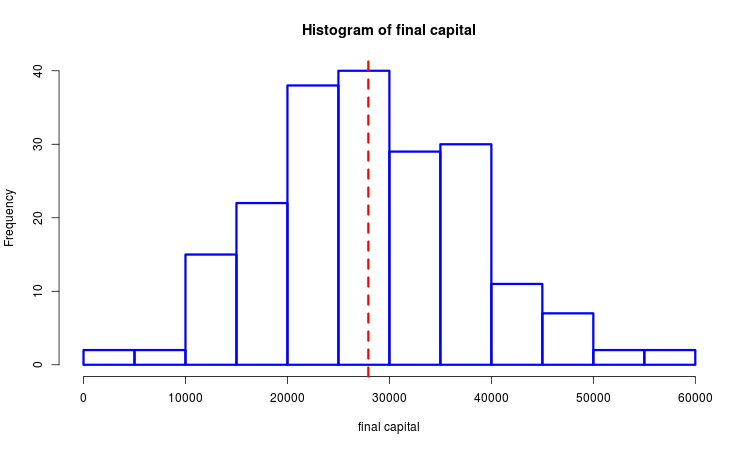```double mean_sd(double &k[])
{
double km[],cn[NSAMPLES];
int nk=ArraySize(k);
ArrayResize(km,nk);
for(int n=0; n<NSAMPLES;++n)
{
sample(k,km);
cn[n]=1.0;
for(int i=0; i<nk;++i) cn[n]*=km[i];
cn[n]-=1.0;
}
return MathMean(cn)/MathStandardDeviation(cn);
}

double med_intq(double &k[])
{
double km[],cn[NSAMPLES];
int nk=ArraySize(k);
ArrayResize(km,nk);
for(int n=0; n<NSAMPLES;++n)
{
sample(k,km);
cn[n]=1.0;
for(int i=0; i<nk;++i) cn[n]*=km[i];
cn[n]-=1.0;
}
ArraySort(cn);
return cn[(int)(0.5*NSAMPLES)]/(cn[(int)(0.75*NSAMPLES)]-cn[(int)(0.25*NSAMPLES)]);
}
```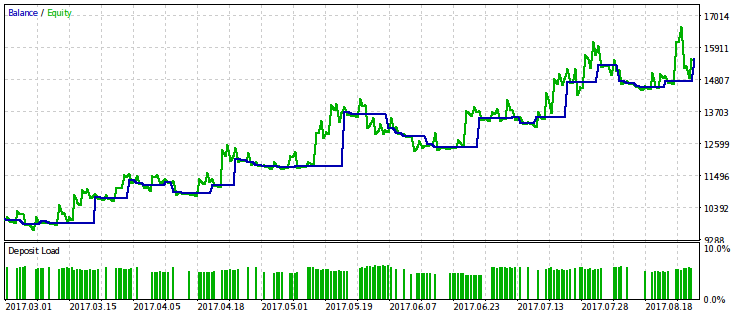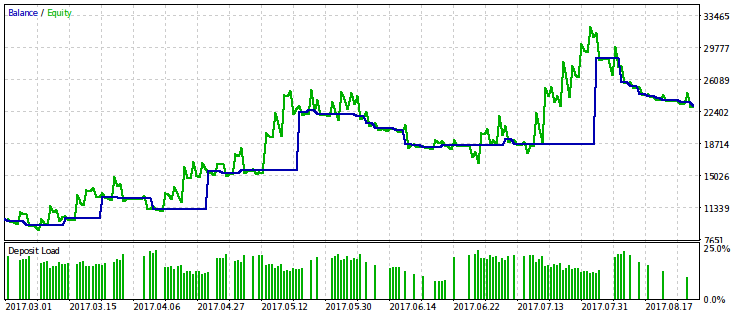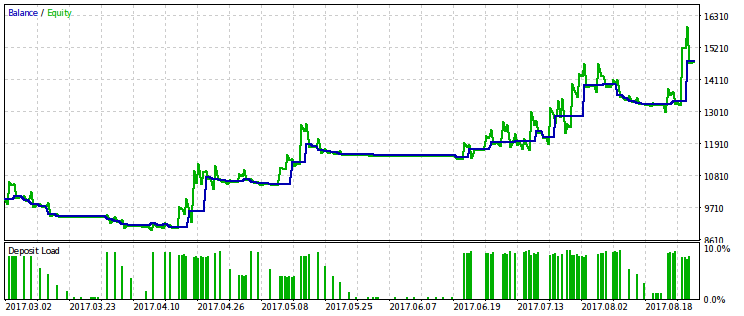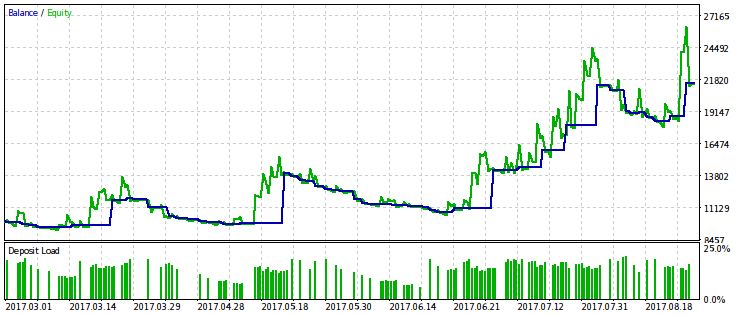### 利润鲁棒性对回撤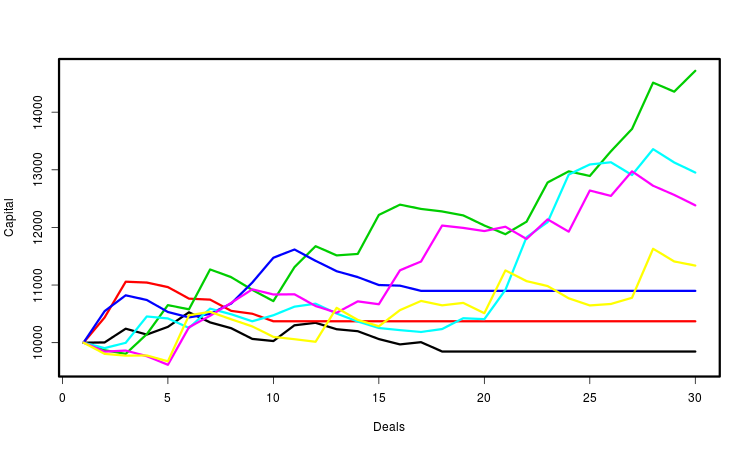```double rmnd_abs(double &k[])
{
if (rmndmin<=0.0||rmndmin>=1.0) return 0.0;
double km[],cn[NSAMPLES];
int nk=ArraySize(k);
ArrayResize(km,nk);
for(int n=0; n<NSAMPLES;++n)
{
sample(k,km);
cn[n]=1.0;
for(int i=0; i<nk;++i)
{
cn[n]*=km[i];
if(cn[n]<rmndmin) break;
}
cn[n]-=1.0;
}
return MathMean(cn);
}

double rmnd_rel(double &k[])
{
if (rmndmin<=0.0||rmndmin>=1.0) return 0.0;
double km[],cn[NSAMPLES],x;
int nk=ArraySize(k);
ArrayResize(km,nk);
for(int n=0; n<NSAMPLES;++n)
{
sample(k,km);
x=cn[n]=1.0;
for(int i=0; i<nk;++i)
{
cn[n]*=km[i];
if(cn[n]>x) x=cn[n];
else if(cn[n]/x<rmndmin) break;
}
cn[n]-=1.0;
}
return MathMean(cn);
}
```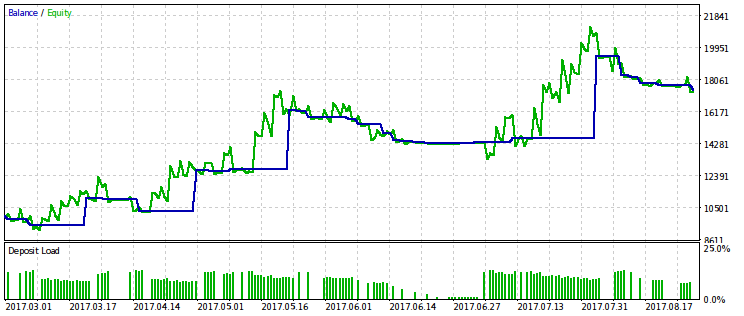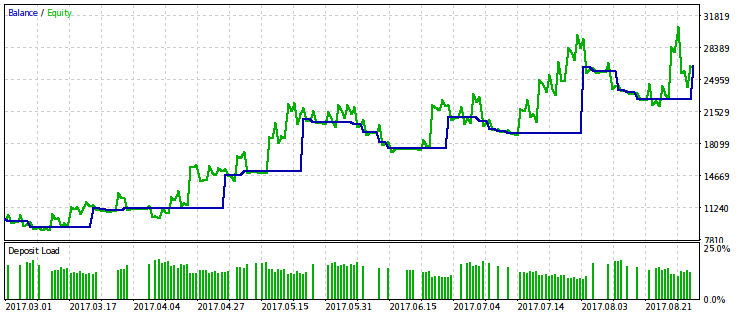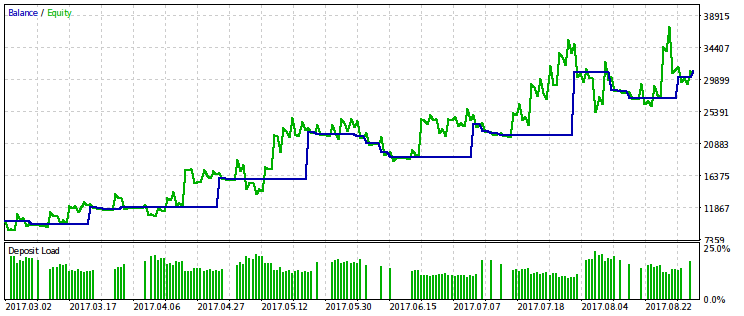rmndmin 的值越小，回撤就越不明显，而优化的结果和通常的利润最大化就越接近，这可以被认为是大数定律的结果——概率论原理。在图像上，这种效果并不是立即被注意到的，因为它们通过垂直轴具有不同的尺度（由于所有图像到相同维度的调整）。另外，因为减小 rmndmin 值而增加的回撤没有立即出现。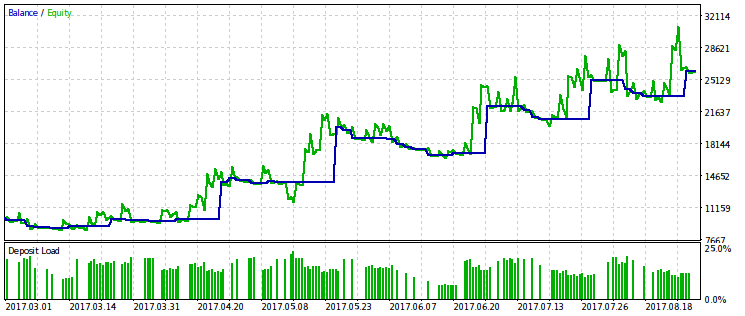### 利润分布的鲁棒性对价格不稳定性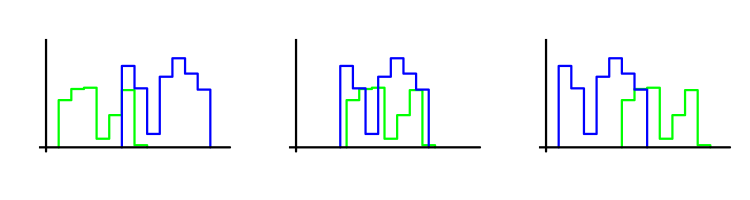```double frw_wmw(double &k[])
{
if (fwdsh<=0.0||fwdsh>=1.0) return 0.0;
int nk=ArraySize(k), nkf=(int)(fwdsh*nk), nkp=nk-nkf;
if(nkf<NDEALSMIN||nkp<NDEALSMIN) return 0.0;
double u=0.0;
for (int i=0; i<nkp; ++i)
for (int j=0; j<nkf; ++j)
if(k[i]>k[nkp+j]) ++u;
return 1.0-MathAbs(1.0-2.0*u/(nkf*nkp));
}

double frw_wmw_prf(double &k[])
{
int nk=ArraySize(k);
double prf=1.0;
for(int n=0; n<nk; ++n) prf*=k[n];
prf-=1.0;
if(prf>0.0) prf*=frw_wmw(k);
return prf;
}
```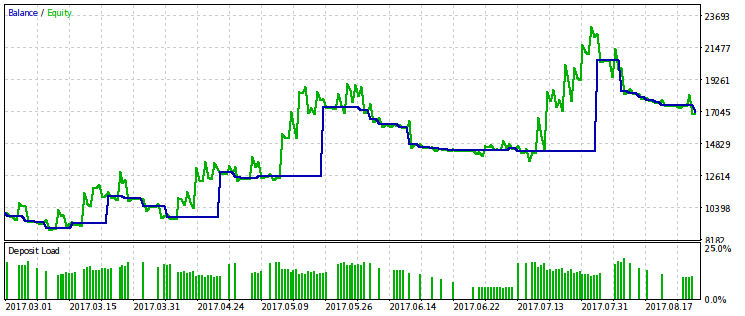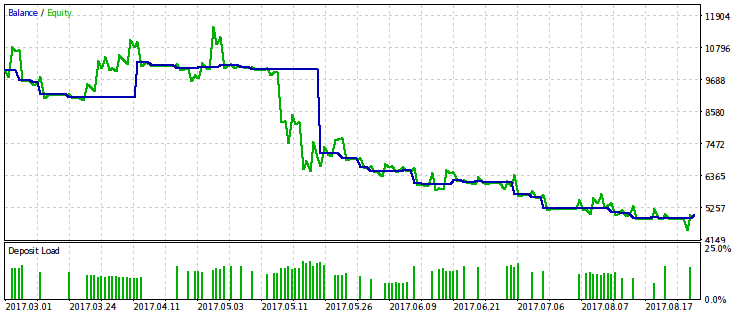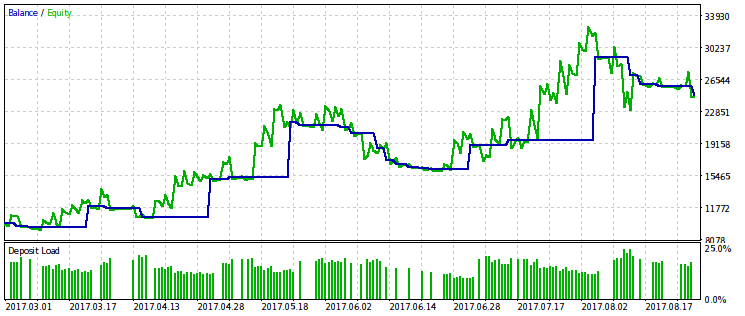### 结论

• 在文章中构建的优化标准可以帮助形式化关于最有效的交易顺序的直觉想法，当从大量选项中选择时，这是有用的。
• 有我们已知的风险衡量方法, 例如VaR (Value At Risk, 风险值) 或者破产概率。如果您在优化标准中直接使用它们，结果将会很糟。交易量和交易的数量将会被低估。因此，需要一个折衷的标准，这取决于两个变量-风险和利润。问题是，我们应该选择哪个标准的版本，因为这两个变量的函数是无限的。例如，可以根据交易策略“激进性”的水平来选择该标准，其差别很大。
• 不同的优化标准的测量稳定性可以是有用的不同的EA种类，例如，跟随趋势的EA将不同于在一个范围内交易的EA，而具有固定获利利润的系统将不同于使用跟踪止损来关闭交易的系统。这样 EA 的利润分布也将属于不同的种类，根据这一点，一个或另一个标准将会更加合理。
• 有些标准对于比较不同的 EA 交易比比较在固定时间段内行为相同而参数集不同的相同 EA 更加有用，而在这样标准的帮助下，就可能比较单一的 EA 交易在不同时间段的工作。
• 蒙特卡洛建模将更加完整，如果有可能通过不仅现有的价格历史，而且它的随机改变的副本到EA的输入。这将允许我们更深入地研究EA的稳定性，尽管以增加计算量为代价。

### 附件中的文件

#

类型 描述
1
Moving Average_mcarlo.mq5 脚本

2
mcarlo.mqh 头文件

mcarlo.mqh (6.8 KB)

#### 该作者的其他文章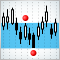10 种横盘交易策略的比较分析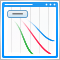改进面板：增加透明化、改变背景色以及继承于 CAppDialog/CWndClient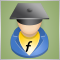在 EA 交易代码中实现指标的计算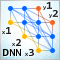深度神经网络 (第六部分)。 神经网络分类器的融合: 引导聚合